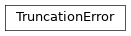# TruncationError¶

Inheritance DiagramMethods

 TruncationError.__init__([eps, ov]) Initialize self. Return a copy of self. TruncationError.from_S(S_discarded[, norm_old]) Construct TruncationError from discarded singular values. TruncationError.from_norm(norm_new[, norm_old]) Construct TruncationError from norm after and before the truncation.

Class Attributes and Properties

 TruncationError.ov_err Error 1.-ov of the overlap with the correct state.
class tenpy.algorithms.truncation.TruncationError(eps=0.0, ov=1.0)[source]

Bases: object

Class representing a truncation error.

The default initialization represents “no truncation”.

Warning

For imaginary time evolution, this is not the error you are interested in!

Parameters
• eps (float) – See below.

• ov (float) – See below.

eps

The total sum of all discared Schmidt values squared. Note that if you keep singular values up to 1.e-14 (= a bit more than machine precision for 64bit floats), eps is on the order of 1.e-28 (due to the square)!

Type

float

ov

A lower bound for the overlap $$|\langle \psi_{trunc} | \psi_{correct} \rangle|^2$$ (assuming normalization of both states). This is probably the quantity you are actually interested in. Takes into account the factor 2 explained in the section on Errors in the TEBD Wikipedia article <https://en.wikipedia.org/wiki/Time-evolving_block_decimation>.

Type

float

Examples

>>> TE = TruncationError()
>>> TE += tebd.time_evolution(...)  # add eps, multiply ov

copy()[source]

Return a copy of self.

classmethod from_norm(norm_new, norm_old=1.0)[source]

Construct TruncationError from norm after and before the truncation.

Parameters
• norm_new (float) – Norm of Schmidt values kept, $$\sqrt{\sum_{a kept} \lambda_a^2}$$ (before re-normalization).

• norm_old (float) – Norm of all Schmidt values before truncation, $$\sqrt{\sum_{a} \lambda_a^2}$$.

classmethod from_S(S_discarded, norm_old=None)[source]

Construct TruncationError from discarded singular values.

Parameters
• norm_old (float) – Norm of all Schmidt values before truncation, $$\sqrt{\sum_{a} \lambda_a^2}$$. Default (None) is 1.
property ov_err
Error 1.-ov of the overlap with the correct state.